# Paul Khuong mostly on Lisp

## Fri, 29 Jul 2011

### An implementation of (one of) Nesterov's accelerated gradient method

I’m a PhD student at Université de Montréal. Contrary to what I suppose most people would expect from the contents of this blog, I don’t (officially) work on compilers. Instead, I chose to focus on mathematical optimisation; specifically, large-scale combinatorial optimisation. Although I sometimes wonder if I made the right choice, the basic rationale is that I’m hoping to contribute to both the compilers and optimisation worlds by bringing them closer.

Sketching algorithms makes up a large fraction of my work, and, lately, Matlisp has been very useful. If you work with algorithms that depend on numerical tools like the BLAS, LAPACK, FFTs, or even just special function like `erf `or `gamma`, you too might like it. In addition to foreign function wrappers and a couple f2cled routines, Matlisp includes a nice reader macro for matrices, and a few convenience functions to manipulate them. Plus, it’s reasonably efficient: it doesn’t try to roll its own routines, can use platform-specific libraries for BLAS and LAPACK, and passes Lisp vectors without copying. It doesn’t look like it was written with threads in mind, unfortunately, but it’s always been good enough for me so far.

The latest project in which I’ve used Matlisp is the prototype implementation of an accelerated gradient method for composite functions [PDF]). The sequel will more or less closely follow the code in my implementation.

I think the program makes a useful, small and self-contained example of how Matlisp can be used; if you need to minimise something that fits in the method’s framework, it might even work well.

### 1 An accelerated gradient method for composite functions

#### 1.1 The theory

In his paper on gradient methods for composite functions, Nesterov describes a family of efficient algorithms to minimise convex functions of the form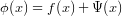where f is a “nice” black box, and Ψ not as nice, but better-known.

Here, “efficient” means that, in addition to being a gradient method (so each iteration is fairly inexpensive computationally), the method converges at a rate of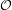(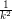), where k is the number of iterations.

f is nice because it must be convex, continuous and differentiable, with a Lipschitz-continuous gradient (continuous and also not too variable). Fortunately, the Lispchitz constant itself may not be known a priori. On the other hand, we only assume that we have access to oracles to compute its value and gradients at arbitrary points.

Ψ simply has to be convex. However, in addition to value and (sub)gradient oracles, we also assume the existence of an oracle to solve problems of the form (with s,a > 0)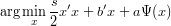Ψ can, for instance, be used to express domain constraints or barriers. In the former case, the last oracle amounts to solving a projection problem.

#### 1.2 The programming interface

My implementation exposes four generic functions to implement one’s own f and Ψ.

`value `and `grad `both take a function description as the first argument, and a point (a Matlisp column vector) as the second argument. They return, respectively, the value and the gradient of the function at that point.

`project `takes a Ψ function description, a distance function description and the multiplicative factor factor a as arguments; the distance functionxx + bx is represented by a `distance `struct with three slots: `n `(the size of the vectors), `s `and `b`. It returns the minimiser ofxx + bx + aΨ(x).

An additional generic function `map-gradient `is also exposed to solve problems of the type arg minxg(x-y) +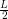x-y2 + Ψ(x), but the default method re-formulates this expression in terms of `project`.

Computing the minimiser of a distance function (without any Ψ term) is a common operation, so it’s exposed as `distance-min`.

The rest of the code isn’t really complicated, but the best explanation is probably the original paper (n.b. although the definitions always come before their use, the distance separating them may be surprisingly large).

Hooks are available to instrument the execution of the method and, e.g., count the number of function or gradient evaluations, or the number of projections. It should be obvious how to use them.

#### 1.3 Example implementations of Ψ

##### 1.3.1 `:unbounded `function

The simplest Ψ is probably Ψ(x) = 0. The accelerated gradient method is then a gradient method for regular differentiable convex optimisation.

`value `and `grad `are trivial.

`project `isn’t much harder: a necessary and sufficient condition for minimisingxx + bx is sx* + b = 0, so x* =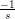b.

##### 1.3.2 `:non-negative `function

To constrain the feasible space to non-negative vectors, we only have to let Ψ(x) = 0 if x 0 (for every component), and Ψ(x) = otherwise.

Again, `value `and `grad `are trivial: `project `will ensure the method only works with non-negative x, and any subgradient suffices.

As for `project`, a little algebra shows that it’s equivalent to finding the feasible x that minimises the distance with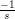b (that’s actually true for any Ψ that represents contraints). For the non-negative orthant, we only have to clamp each negative component of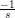b to 0:

```(defun clamp (matrix)
(map-matrix! (lambda (x)
(declare (type double-float x))
(max x 0d0))
matrix))

(defmethod project ((psi (eql :non-negative)) distance Ak)
(declare (type distance distance)
(ignore Ak))
(clamp (distance-min distance)))```

#### 1.4 A sample “nice” function f: linear least squares

The linear least squares problem consists of finding a vector x that minimizes (the multiplication by half is just to get a nicer derivative)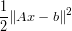This kind of problem can come up when fitting a line or a hyperplane to a set of observations.

`value `is straightforward:

```(defmethod value ((lls linear-least-square) x)
;; A set of convenient matrix operation is available
(let ((diff (m- (m* (lls-A lls) x)
(lls-b lls))))
(* .5d0 (ddot diff diff)))) ; ddot ~= a call to MATLISP:DOT```

A little algebra shows that the gradient can be computed with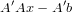The constructor `make-lls `precomputes AA and A, so we get

```(defmethod grad ((lls linear-least-square) x)
;; we can also call BLAS routines directly
(axpy! -1 (lls-Atb lls)
(m* (lls-AtA lls) x)))```

LAPACK has built-in functions to solve that problem, for example `gelsy `(that’s on my puny macbook air):

```;; Create an LLS instance with 2000 rows (observations)
;; and 400 columns (variables).
AGM> (defparameter *lls* (make-random-lls 2000 400))
*LLS*
AGM> (time (gelsy (lls-a *lls*) (lls-b *lls*) (* 1000 (expt 2d0 -52))))
Evaluation took:
0.829 seconds of real time
0.827092 seconds of total run time (0.806912 user, 0.020180 system)
[ Run times consist of 0.036 seconds GC time, and 0.792 seconds non-GC time. ]
99.76% CPU
1,323,883,144 processor cycles
6,425,552 bytes consed

#<REAL-MATRIX  400 x 1
-2.20134E-2
1.92827E-2
-7.19030E-3
-4.01685E-2
:
7.19374E-3 >
400
AGM> (value *lls* *)
66.5195278164384d0```

Although we have very solid specialised methods to solve least squares problems, it makes for a nice non-trivial but easily-explained example.

```;; 400 variables, with an LLS instance for f and
;; a default Psi of :unbounded
AGM> (time (solve (make-agm-instance 400 *lls*)))
Evaluation took:
32.426 seconds of real time
31.266698 seconds of total run time (31.003772 user, 0.262926 system)
[ Run times consist of 0.799 seconds GC time, and 30.468 seconds non-GC time. ]
96.43% CPU
51,779,907,728 processor cycles
795,491,552 bytes consed

66.51952785790199d0
#<REAL-MATRIX  400 x 1
-2.20108E-2
1.92838E-2
-7.19136E-3
-4.01690E-2
:
7.19797E-3 >
[...]```

Pretty horrible, even more so when we add the 0.9 seconds it takes to precompute Aand AA.

Here’s an interesting bit:

```;; Use the optimal value as the starting point
AGM> (time (solve (make-agm-instance 400 *lls* :x0 (second /))))
Evaluation took:
0.043 seconds of real time
0.043683 seconds of total run time (0.043445 user, 0.000238 system)
102.33% CPU
69,793,776 processor cycles
1,525,136 bytes consed

66.51952785758927d0
#<REAL-MATRIX  400 x 1
-2.20108E-2
1.92838E-2
-7.19136E-3
-4.01690E-2
:
7.19795E-3 >
[...]```

This method naturally exploits warm starts close to the optimal solution…useful when you have to solve a lot of similar instances.

Another interesting property is shown here:

```AGM> (time (solve (make-agm-instance 400 *lls*)
Evaluation took:
1.961 seconds of real time
[...]
66.52381619339305d0 ; delta: 1/2.3e2
[...]
AGM> (time (solve (make-agm-instance 400 *lls*)
Evaluation took:
7.328 seconds of real time
[...]
66.51957600391108d0 ; delta: 1/2.1e4
[...]
AGM> (time (solve (make-agm-instance 400 *lls*)
Evaluation took:
19.275 seconds of real time
[...]
66.51952840584295d0 ; delta: 1/1.7e6
[...]
AGM> (time (solve (make-agm-instance 400 *lls*)
Evaluation took:
31.146 seconds of real time
[...]
66.51952785790199d0 ; delta: 1/2.4e7
```

The run time (and the number of iteration or function/gradient evaluation) scales nicely with the precision.

#### 1.5 Efficiency

The implementation wasn’t written with efficiency in mind, but rather to rapidly get a working prototype. However, nearly all the cost is incurred by calls to the BLAS. If performance were an issue, I’d mostly focus on minimising consing and copies by reusing storage and calling (destructive versions of) BLAS functions to fuse operations.

posted at: 00:19 | /Lisp/Math | permalinkContact me by email: pvk@pvk.ca.